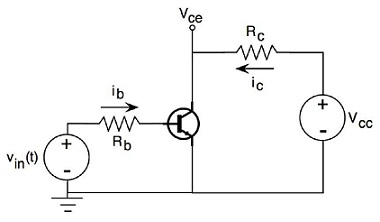### The given image compute what you would expect for vce

Assignment Help Electrical Engineering
##### Reference no: EM13993721

If β=100, and Vin=0.2cos(2πf*t)+2 V where f=100 Hz, compute what you would expect for Vce. Assume Vcc=5V, Rb=20 kΩ, Rc=200 Ω from the given image.#### Find (a) the equivalent inductance and (b) the total energy

A 16-mH inductor and a 15-mH inductor are connected in series with a 1-A current source. Find (a) the equivalent inductance and (b) the total energy stored.

#### Write the c statement needed to arm the port j

1. Write the C statement needed to arm the Port J interrupt? 2. What does placing asm("cli") at the beginning of an ISR do? 3. Explain what the software interrupt (SWI) does.

#### What is the sinusoidal expression for the voltage

The current through a 20-ohm inductive reactance is given. What is the sinusoidal expression for the voltage? Sketch the (v) and (i) sinusoidal waveforms on the same axis. A -

#### The rated power for the primary side be supported bythe

With this particular connection can the rated power for the primary side be supported bythe secondary side? If not, why and how could the transformer be changed to supportrate

#### Identify an electrical component either ac or dc

In this discussion activity, address the following in your primary, original posting: Identify an electrical component either AC or DC and clearly describe how it works and w

#### Sketch the transfer characteristic

Consider the circuit below containing two ideal (i.e., zero forward voltage drop) Zener diodes whose breakdown voltage is specified. Sketch the transfer characteristic (Vout v

#### Calculate volumetric efficiency and net thermal efficiency

Consider the case of boosting for this engine and plot volumetric efficiency, netthermal efficiency, and residual gas fraction as a function of the ratio of intake toexhaust

#### Determine the voltage gain for the ce

Answer the following questions:1. Determine the voltage gain for the CE ampli?er in the following figure.2. If a load resistance of 600 ? is placed on the output of the ampli?

### Write a Review